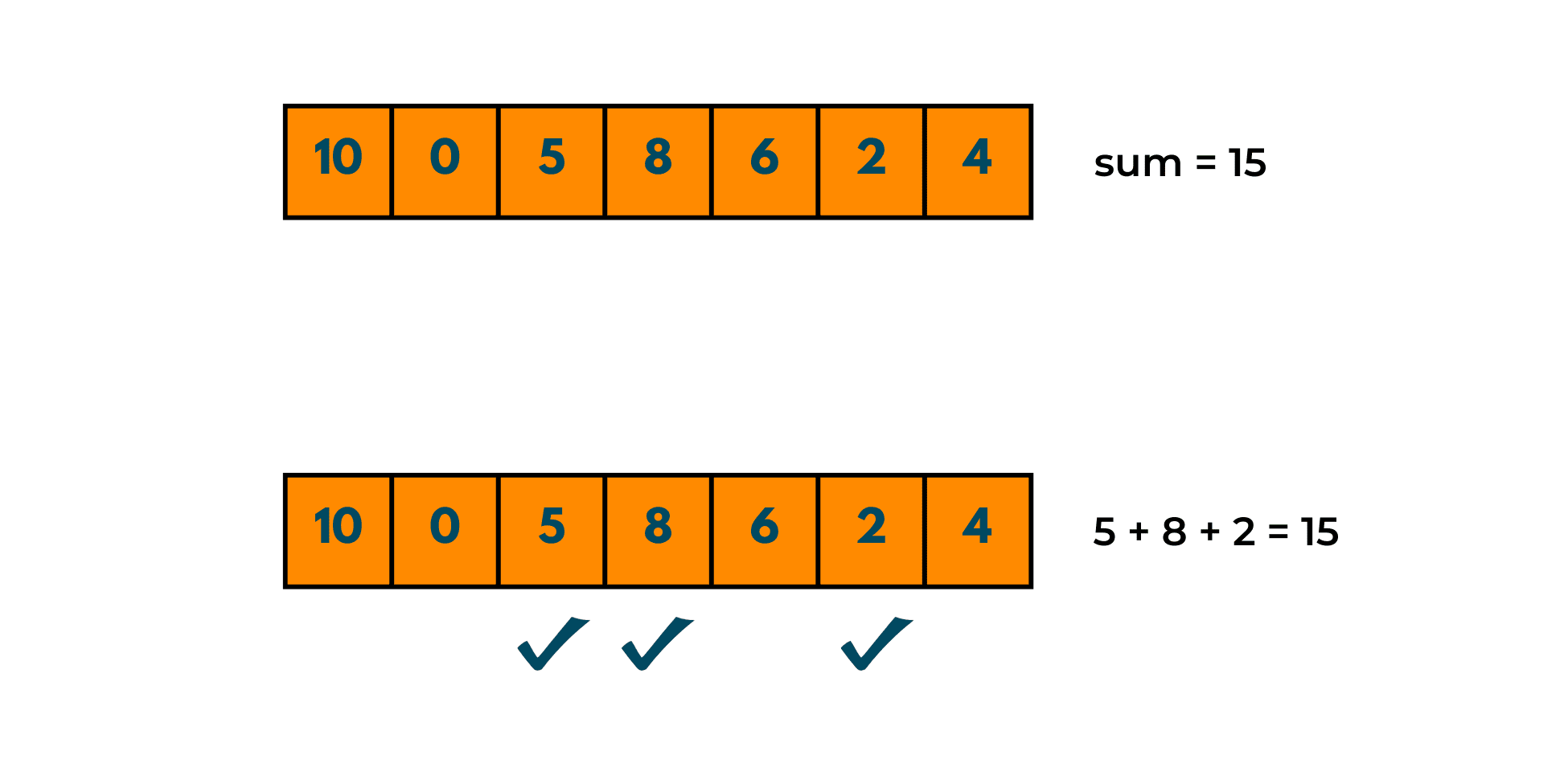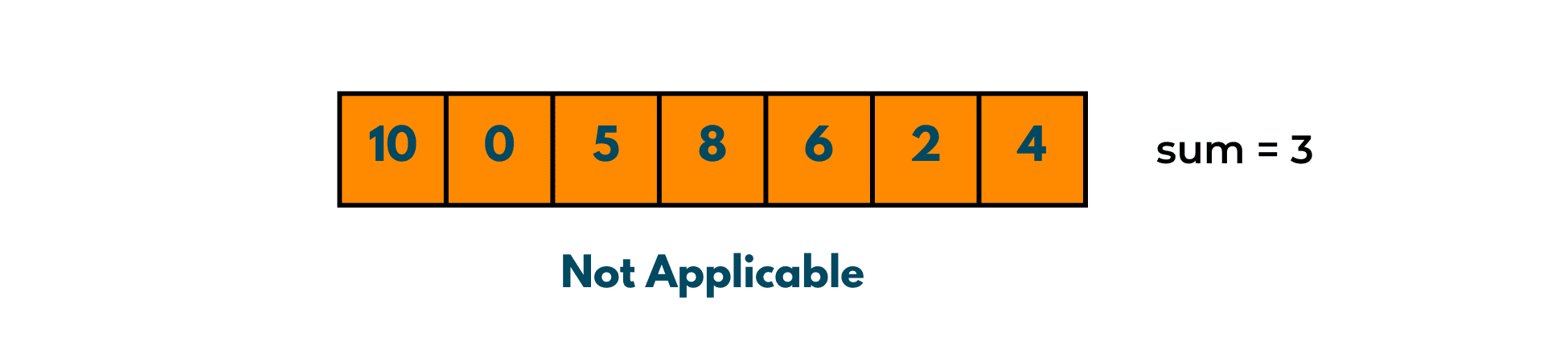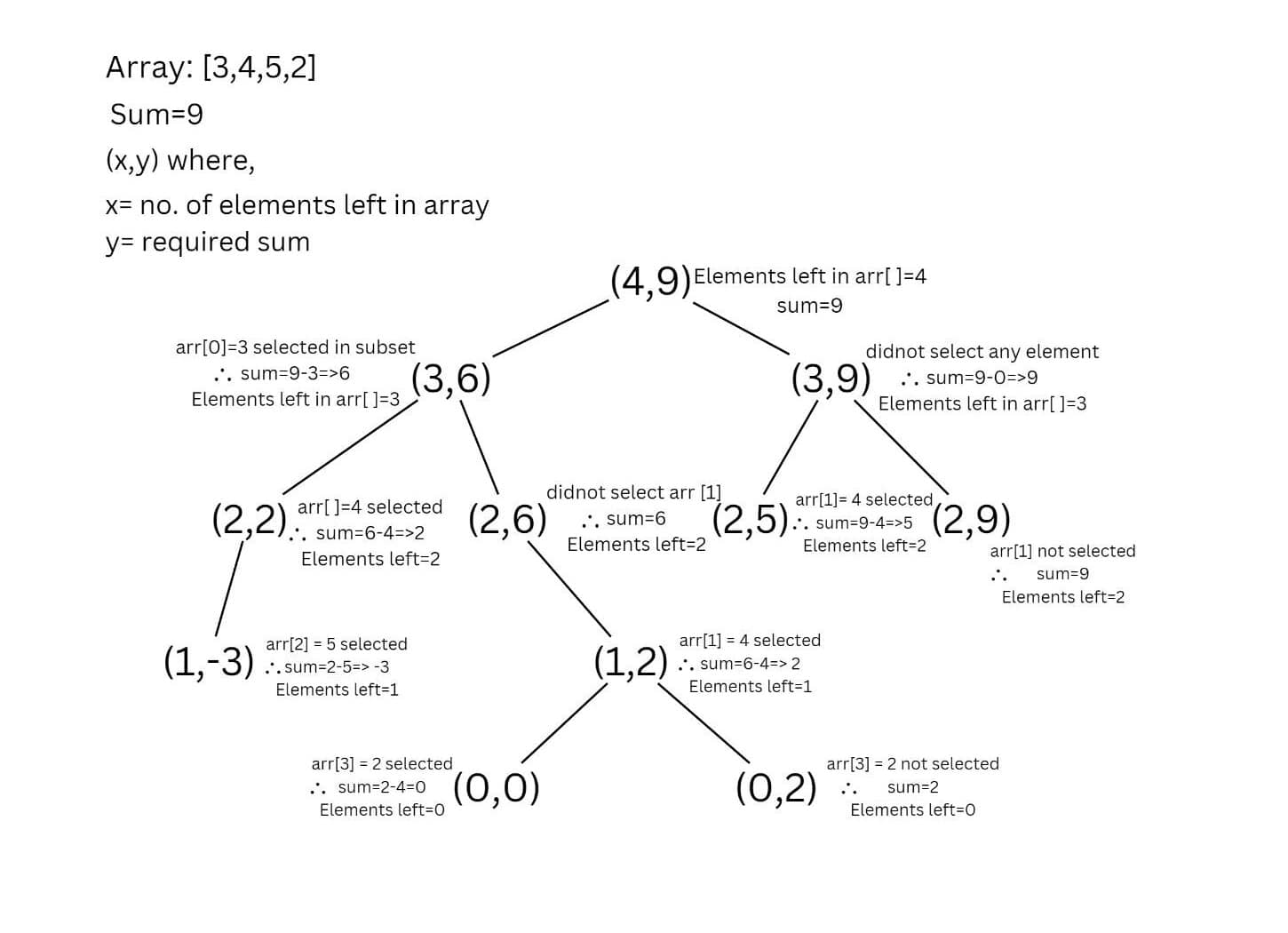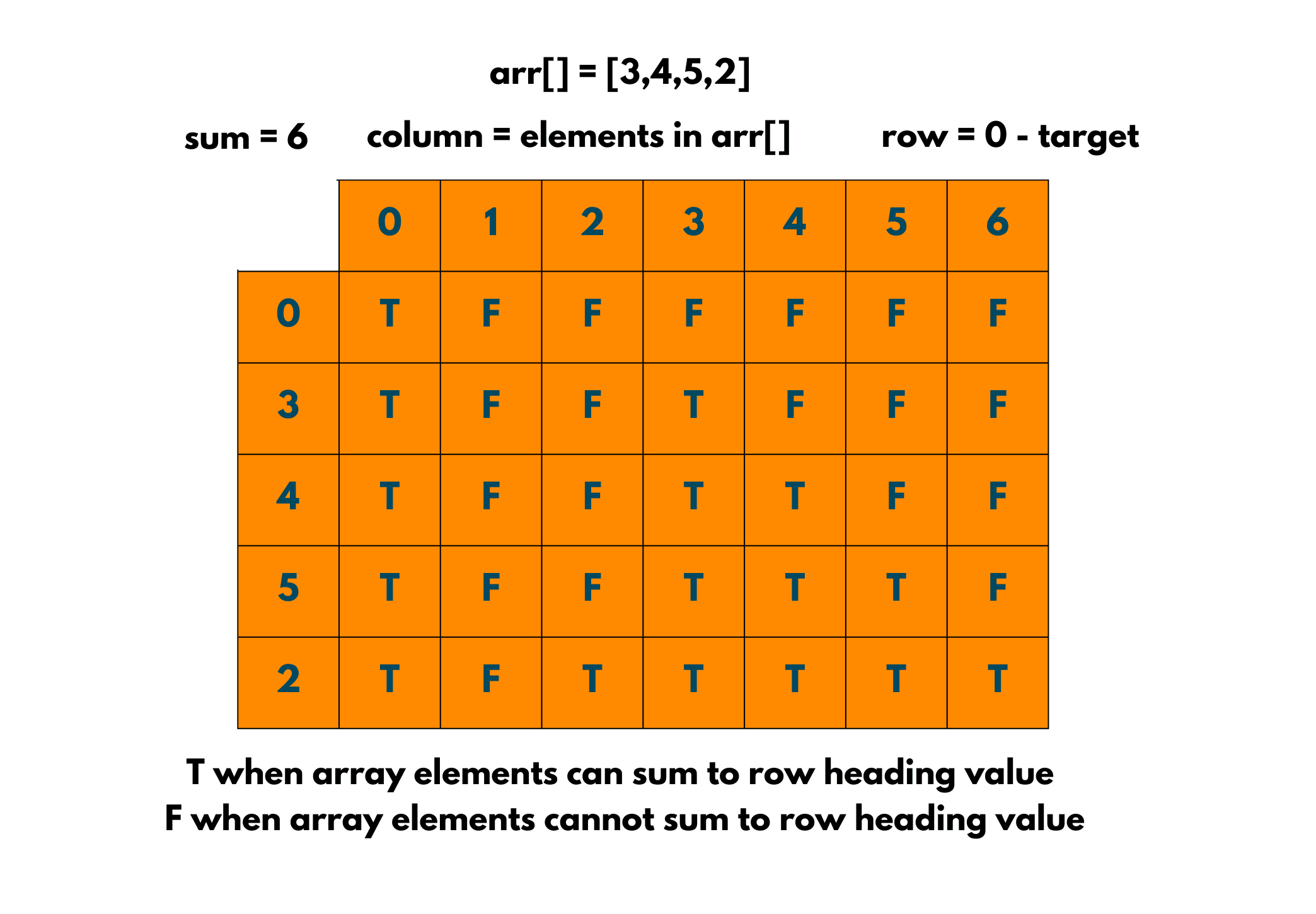# Subset Sum Problem Explained (Dynamic Programming)

With thousands of students preparing for jobs in the technology industry, the best way for you to stand out is to practice as many questions as you can. Sometimes even complex questions can be solved very easily with just a little trick and sometimes the interviewer is not looking for an optimal solution but just the right approach.

So, in today's blog, we present to you a question that is commonly asked by tech giants like Microsoft and Amazon. Yes, we're talking about the Subset Sum Problem!

We understand that code can be pasted from anywhere, the right thing to build in today's engineering students is the intuition and approach behind the question. We'll walk you through the question and give you awesome tricks to solve it.

From naive solutions to solving questions using advanced concepts like Dynamic Programming, we're going to do it with you!

## What is the Subset Sum Problem?

Let's look at the problem statement: "You are given an array of non-negative numbers and a value 'sum'. You have to find out whether a subset of the given array is present whose sum is equal to the given value."

Let's look at an example:

Input: {10, 0, 5, 8, 6, 2, 4}, 15

Output: True

Explanation: The sum of the subset {5,8,2} gives the sum as 15. Therefore, the answer comes out to be true.Let's take a look at another example for your clarification.

Input: {10, 0, 5, 8, 6, 2, 4}, 3

Output: False

Explanation: There is no subset whose sum is equal to the given value. Hence, the output is false.## Understanding the Problem

Before moving towards any method, you need to make sure whether you understood the Subset Sum Problem correctly or not. In interviews also, even if you do not know the whole code, you can still conquer it by moving in the right direction and following the right approach. So, what exactly is being asked over here?

If you are familiar with the concepts of subset then this question is not that difficult for you to understand. What is a subset? A subset is a set that contains the elements of a previously defined set. For eg. {a, b} is a subset of {a,b,c,e,f}. In this question also, you have to find a subset or the set of numbers from the given array that amount to the given value in the input.

Now that you have understood the problem, let's move on to some methods with which you can solve the problem.

## How to Solve the Sum of Subset Problem?

Let's look at 3 best methods to solve it:

### Method 01) Recursion

Recursion is a widely known concept and is majorly famous in questions where you need to process the same data again and again till you hit the base condition. So, in this method, we'll be using recursion only. Let's understand the algorithm and try to decode the question with some illustrations.

Algorithm:

1. Take input of the array and the value 'sum'.
2. Find the size of the array.
3. Create a function whose return type is boolean so that it returns True if the sum is found otherwise False.
4. In this function, check whether the last element of the array is greater than the 'sum' or not.
5. If it is greater then skip to the next element and if not, consider it in the subset.
6. Keep decreasing the value of the sum by subtracting the value of the last element (if considered).
7. Keep the base condition to be true till the elements are present and till the given sum is not equal to 0.

Recursive Formula and Base Cases for the Code:

```SubsetSum(arr, size, sum) = SubsetSum(arr, size-1, sum) || SubsetSum(arr, size-1, sum-arr[size-1])
Base Cases:
SubsetSum(arr, size, sum) = false, if sum > 0 and size == 0
SubsetSum(arr, size, sum) = true, if sum == 0
```

Take a look at the given illustration as well!### Method 02) Dynamic Programming

Using a Recursive technique to solve this question is good, but with Dynamic Programming, the time complexity of the solution can be improved by manifolds. The time complexity of the recursive solution is exponential, therefore, the need to come up with a better solution arises. So, look at the given solution that uses the top-down approach to Dynamic Programming.

Algorithm:

1. Take input of the array and the value 'sum'.
2. Find the size of the array.
3. Create a function whose return type is boolean so that it returns True if the sum is found otherwise False.
4. Create a 2d array of size(size+1)*(target+1)
5. Now, this state of the 2d array will be true if the subset of the given sum is found else, it will be false.
6. While traversing the array, if the current element has a value greater than the ‘current sum value’ it will be copied for previous cases.
7. If the current sum value is greater than the ‘ith’ element, we'll observe whether the previous states have experienced the sum=j.

The formula used in the method:

```if (arr[i-1] > j)
DP[i][j] = DP[i-1][j]
else
DP[i][j] = DP[i-1][j] || DP[i-1][j-arr[i-1]]
```### Method 03) Memoization Technique

The Dynamic Programming method solves the question in polynomial time, but what if your interviewer asks you to optimize it further? Well, we got you covered! Using this Memoization Technique, your interviewer will definitely get impressed. Although this method also uses recursion, we optimize it further using the memoization technique.

Algorithm:

1. Take input of the array and the value 'sum'.
2. Find the size of the array.
3. Create a function whose return type is boolean so that it returns True if sum is found otherwise False.
4. Create a 2d matrix and initialize it with -1 or any other negative number.
5. Using the same recursive method as in 1st method, but storing the value of the previous call.

### Code Implementation with C++

Here is the C++ code to solve the Subset Sum Problem:

```#include <bits/stdc++.h>
using namespace std;

//creating a global matrix
int arr;

// function to check whether subset with given sum is present or not
int subsetSum(int a[], int n, int sum)
{
if (sum == 0)
return 1;     //subset with given sum is present

if (n <= 0)
return 0;     //subset with given sum is abset

if (arr[n - 1][sum] != -1)
return arr[n - 1][sum];

//if call of a[n-1] is greater than sum then return the following value
if (a[n - 1] > sum)
return arr[n - 1][sum] = subsetSum(a, n - 1, sum);
else
{
// 2 calls are initiated here since we do not know which condition could be true.
return arr[n - 1][sum] = subsetSum(a, n - 1, sum) || subsetSum(a, n - 1, sum - a[n - 1]);
}
}

int main()
{
//initializing the matrix with -1 or any other negative value.
memset(arr, -1, sizeof(arr));
int n = 5;
int a[] = {1, 5, 3, 7, 4};
int sum = 12;

if (subsetSum(a, n, sum))
{
cout << "YES" << endl;
}
else
cout << "NO" << endl;
}
```

### Code Implementation with Java

Here is the Java code to solve this problem:

```class Solution{

// function to check whether the subset of given sum is present or not
static int subsetSum(int a[], int n, int sum)
{
//Initializing  matrix as -1.
int arr[][] = new int[n + 1][sum + 1];
for (int i = 1; i <= n; i++) {
for (int j = 1; j <= sum; j++) {
arr[i][j] = -1;
}
}

if (sum == 0)
return 1;

if (n <= 0)
return 0;

if (arr[n - 1][sum] != -1)
return arr[n - 1][sum];
if (a[n - 1] > sum)
return arr[n - 1][sum]
= subsetSum(a, n - 1, sum);
else {
if (subsetSum(a, n - 1, sum) != 0
|| subsetSum(a, n - 1, sum - a[n - 1])
!= 0) {
return arr[n - 1][sum] = 1;
}
else
return arr[n - 1][sum] = 0;
}
}

public static void main(String[] args)
{

int n = 5;
int a[] = { 1, 5, 3, 7, 4 };
int value = 12;
if (subsetSum(a, n, value) != 0) {
System.out.println("YES");
}
else
System.out.println("NO");
}
}
```

Output:

```Yes
```

## Comparative Study of Complexity Analysis

Here are the time complexities for all ways to solve the Subset Sum Problem:

 S.No. Method Name Time Complexity Space Complexity 1. Recursion exponential depends upon size of array 2. Dynamic Programming O(sum*size) O(sum*size) 3. Memoization Technique O(sum*size) O(sum*size) + O(size)

## Summing Up

With the above 3 methods, we are sure that you'll be able to analyze and solve the Subset Sum Problem with wonderful time complexity. After reading this tech blog we are sure, you'll have a basic understanding of memoization as well as Dynamic Programming technique.

We'll keep bringing great and informative articles to FavTutor for your betterment.

### FavTutor - 24x7 Live Coding Help from Expert Tutors!